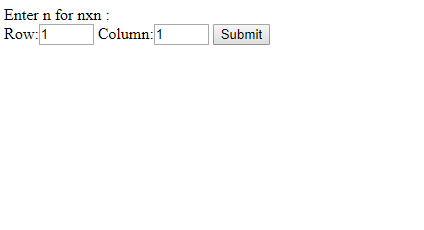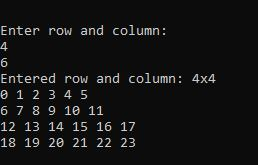# How to take user input for two dimensional (2D) array in PHP ?

• Last Updated : 23 Dec, 2021

There are two methods to take user input in PHP in two dimensional (2D) array.
Approach 1:

• Use HTML forms through PHP GET & POST method to take user input in two dimensional (2D) array.
• First, input data to HTML forms.
• Then use GET or POST method of PHP to get or post those input data into a variables.
• Finally, use those variable which holds input data and process using for loop.
• Though it is form two dimensional array so, you need two indices/variables for processing in for loop.
• Enter input one below another to state as two variables.

Example 1: Below example illustrate how to input user data for 2D-array using Form POST method.

## php

 `"``;``echo` `"
``    ``Row:``    ``Column:``    ````";` `// Submit user input data for 2D array``if` `(isset(``\$_POST``[``'submit'``])) {``    ` `    ``// POST submitted data``    ``\$dimention1` `= ``\$_POST``[``"1d"``];``    ` `    ``// POST submitted data``    ``\$dimention2` `= ``\$_POST``[``"2d"``];``    ` `    ``echo` `"Entered 2d nxn: "` `. ``\$dimention1``            ``. ``"x"` `. ``\$dimention2` `. ``"
"``;``    ``\$d` `= [];``    ``\$k` `= 0;``    ` `    ``for``(``\$row` `= 0; ``\$row` `< ``\$dimention1``; ``\$row``++) {``        ``for` `(``\$col` `= 0; ``\$col` `< ``\$dimention2``; ``\$col``++) {``            ``\$d``[``\$row``][``\$col``]= ``\$k``++;``        ``}``    ``}``    ` `    ``for` `(``\$row` `= 0; ``\$row` `< ``\$dimention1``; ``\$row``++) {``        ``for` `(``\$col` `= 0; ``\$col` `< ``\$dimention2``; ``\$col``++) {``            ``echo` `\$d``[``\$row``][``\$col``].``" "``;``        ``}``        ``echo` `"
"``;``    ``}``}``?>`

Output:Approach 2:

• To take user input in php for two dimensional (2D) by using fopen() function which helps to get user input either at runtime or by external input file.
• First, assign those input data to variables.
• Finally, use those variable which holds input data and process using for loop.
• Though it is form two dimensional array so, you need two indices/variables for processing in for loop.

Example: Below example illustrate how to input user data for 2D array using fopen() function.

## php

 ````

Output:My Personal Notes arrow_drop_up